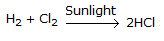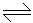# Chemical Engineering - Chemical Reaction Engineering

### Exercise :: Chemical Reaction Engineering - Section 6

1.

The order of the reaction,, is

 A. 0 B. 1 C. 2 D. 3

Explanation:

No answer description available for this question. Let us discuss.

2.

Arhenious equation shows the variation of __________ with temperature.

 A. reaction rate B. rate constant C. energy of activation D. frequency factor

Explanation:

No answer description available for this question. Let us discuss.

3.

When a catalyst increases the rate of chemical reaction, the rate constant

 A. decreases B. increases C. remains constant D. becomes infinite

Explanation:

No answer description available for this question. Let us discuss.

4.

In which of the following reactions, the equilibrium will shift to the right, if the total pressure is increased?

 A. H2 + Cl22HCl B. N2 + O22NO C. N2O42 NO2 D. H2 + I22HI

Explanation:

No answer description available for this question. Let us discuss.

5.

The catalyst in a first order chemical reaction changes the

 A. equilibrium constant B. activation energy C. heat of formation of the product D. heat of reaction